# NCERT Solutions for Class 6 Maths Chapter 7 Fractions Ex 7.5

NCERT Solutions for Class 6 Maths Chapter 7 Fractions Ex 7.5 are part of NCERT Solutions for Class 6 Maths. Here we have given NCERT Solutions for Class 6 Maths Chapter 7 Fractions Ex 7.5.

 Board CBSE Textbook NCERT Class Class 6 Subject Maths Chapter Chapter 7 Chapter Name Fractions Exercise Ex 7.5 Number of Questions Solved 5 Category NCERT Solutions

## NCERT Solutions for Class 6 Maths Chapter 7 Fractions Ex 7.5

Question 1.
Write these fractions appropriately as additions or subtractions: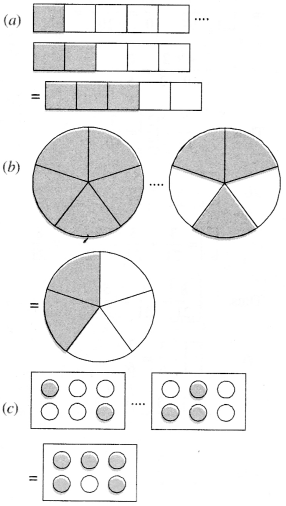Solution :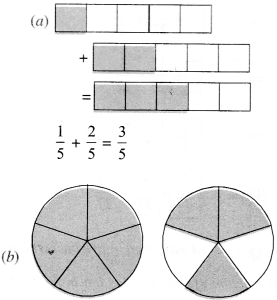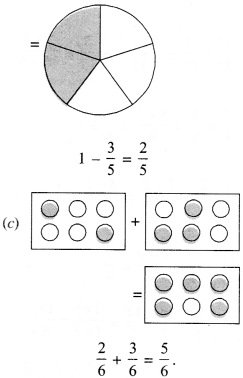Question 2.
Solve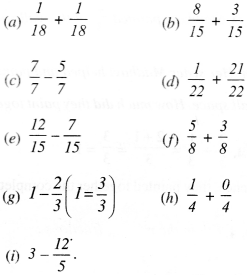Solution :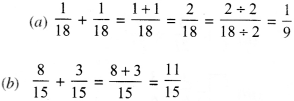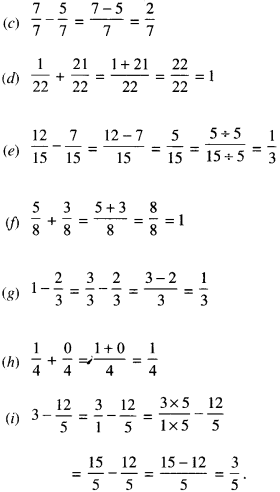Question 3.
Shubham painted $$\frac { 2 }{ 3 }$$ of the wall space in his room. Her sister Madhavi helped and painted $$\frac { 1 }{ 3 }$$ of the wall space. How much did they paint together?
Solution :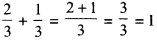Hence, they painted together with the complete wall space. ,

Question 4.
Fill in the missing fractions :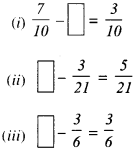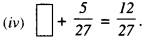Solution :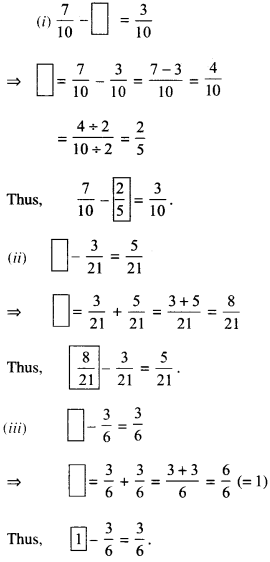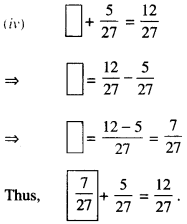Question 5.
Javed was given $$\frac { 5 }{ 7 }$$ of a basket of oranges. What fraction of oranges was left in the basket?
Solution :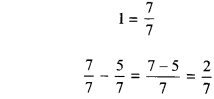Hence, fraction $$\frac { 2 }{ 7 }$$ of oranges was left in the basket.

We hope the NCERT Solutions for Class 6 Maths Chapter 7 Fractions Ex 7.5 help you. If you have any query regarding NCERT Solutions for Class 6 Maths Chapter 7 Fractions Ex 7.5, drop a comment below and we will get back to you at the earliest.